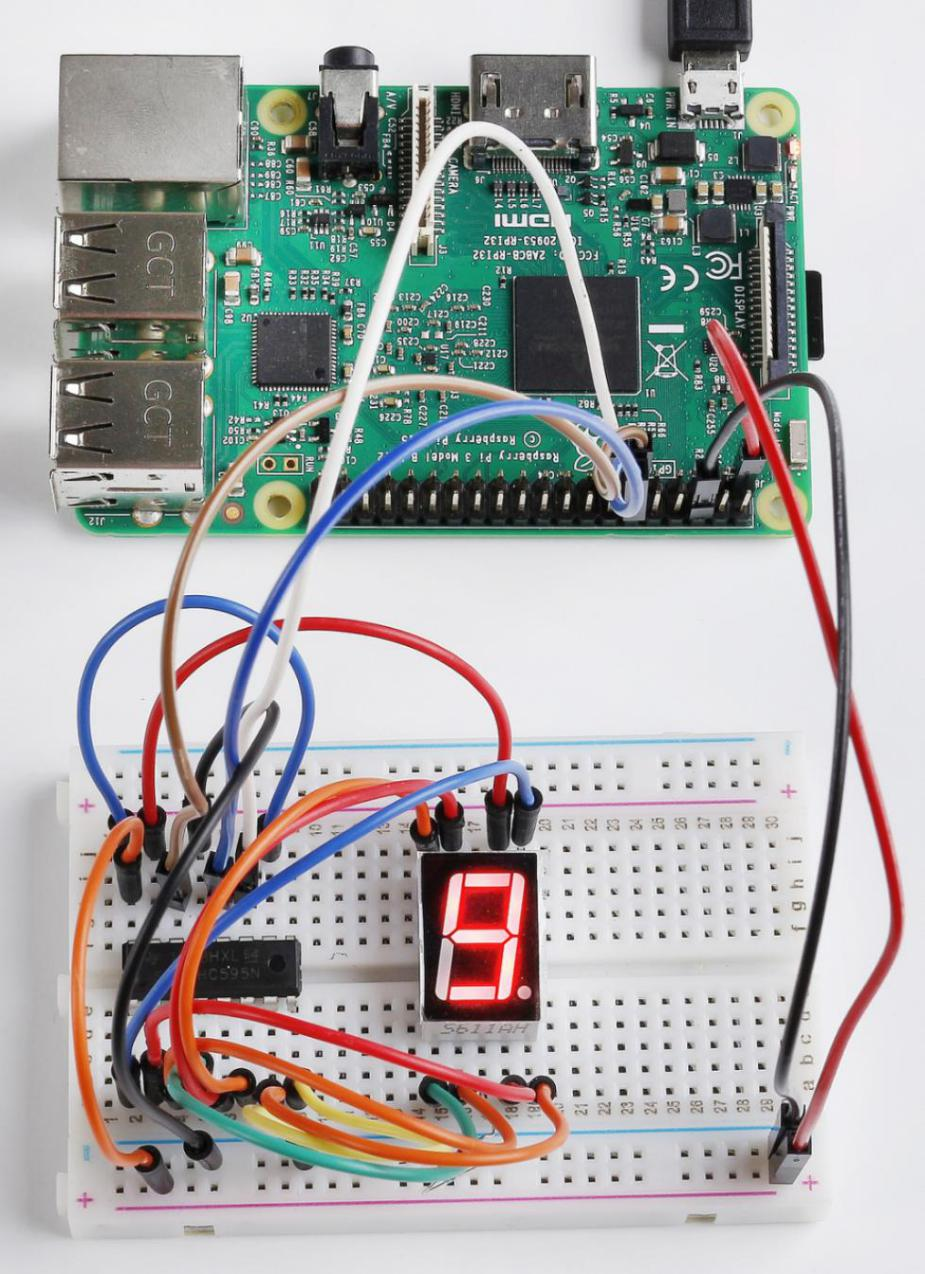# Lesson 19 7-segment¶

## Introduction¶

Generally, there are two ways to drive a single 7-Segment Display. One way is to connect its 8 pins directly to eight ports on the Raspberry Pi. Or you can connect the 74HC595 to three ports of the Raspberry Pi and then the 7- segment display to the 74HC595. In this experiment, we will use the latter. By this way, we can save five ports – considering the board’s limited ports, and this is very important. Now let’s get started!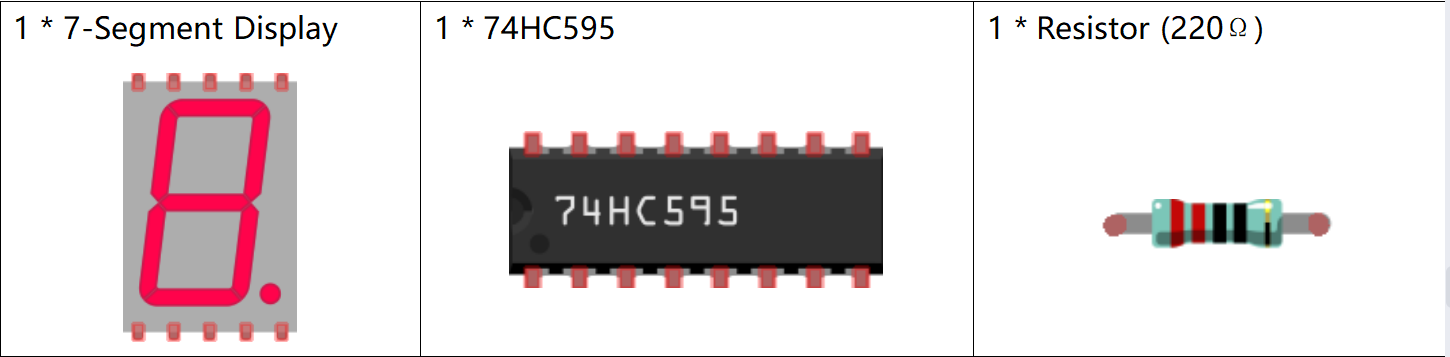## Principle¶

7-Segment Display

A 7-Segment Display is an 8-shaped component which packages 7 LEDs. Each LED is called a segment – when energized, one segment forms part of a numeral to be displayed.

There are two types of pin connection: Common Cathode (CC) and Common Anode (CA). As the name suggests, a CC display has all the cathodes of the 7 LEDs connected when a CA display has all the anodes of the 7 segments connected. In this kit, we use the former.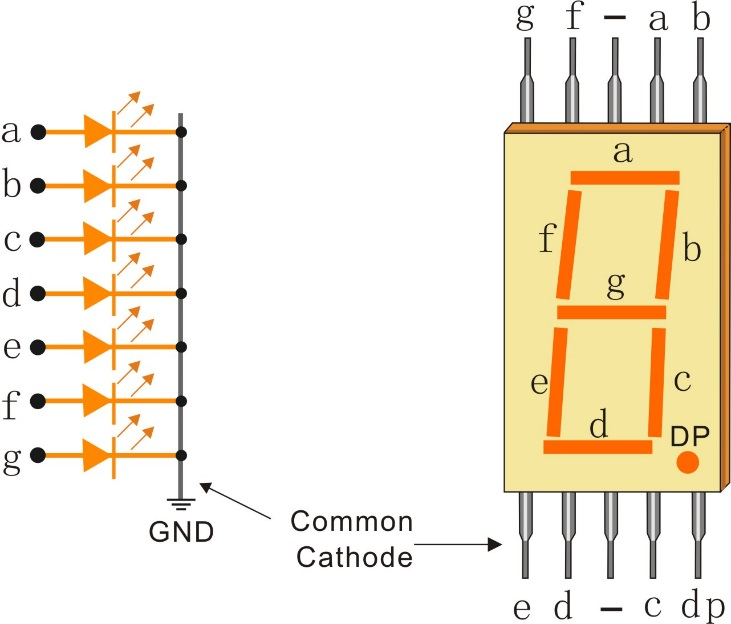Each of the LEDs in the display is given a positional segment with one of its connection pins led out from the rectangular plastic package. These LED pins are labeled from “a” through to “g” representing each individual LED. The other LED pins are connected together forming a common pin. So by forward biasing the appropriate pins of the LED segments in a particular order, some segments will brighten and others stay dim, thus showing the corresponding character on the display.

Display Codes

To help you get to know how 7-Segment Displays(Common Cathode) display Numbers, we have drawn the following table. Numbers are the number 0-F displayed on the 7-Segment Display; (DP) GFEDCBA refers to the corresponding LED set to 0 or 1, For example, 00111111 means that DP and G are set to 0, while others are set to 1. Therefore, the number 0 is displayed on the 7-Segment Display, while HEX Code corresponds to hexadecimal number.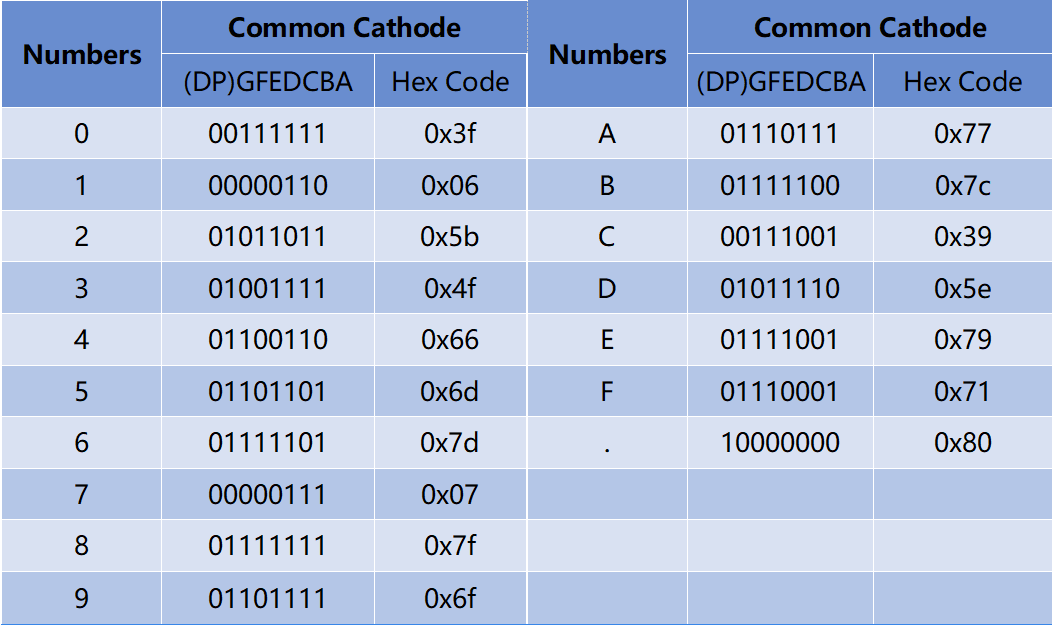## Schematic Diagram¶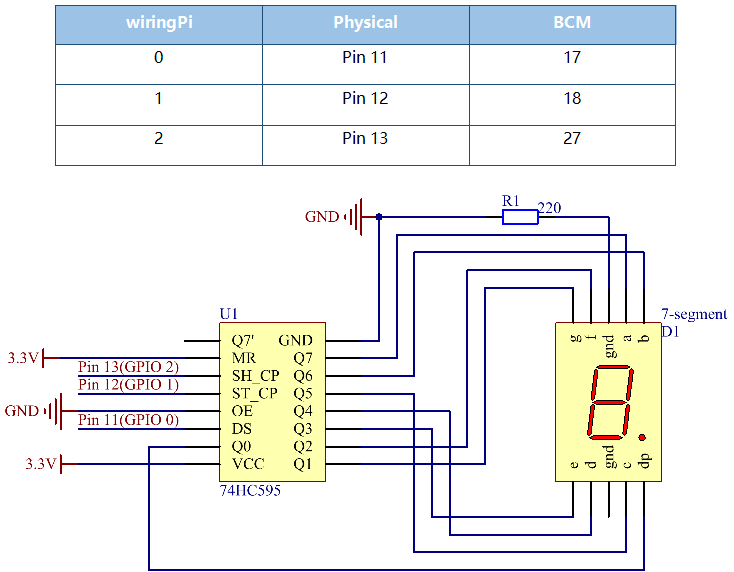## Build the Circuit¶

Note

Recognize the direction of the chip according to the concave on it.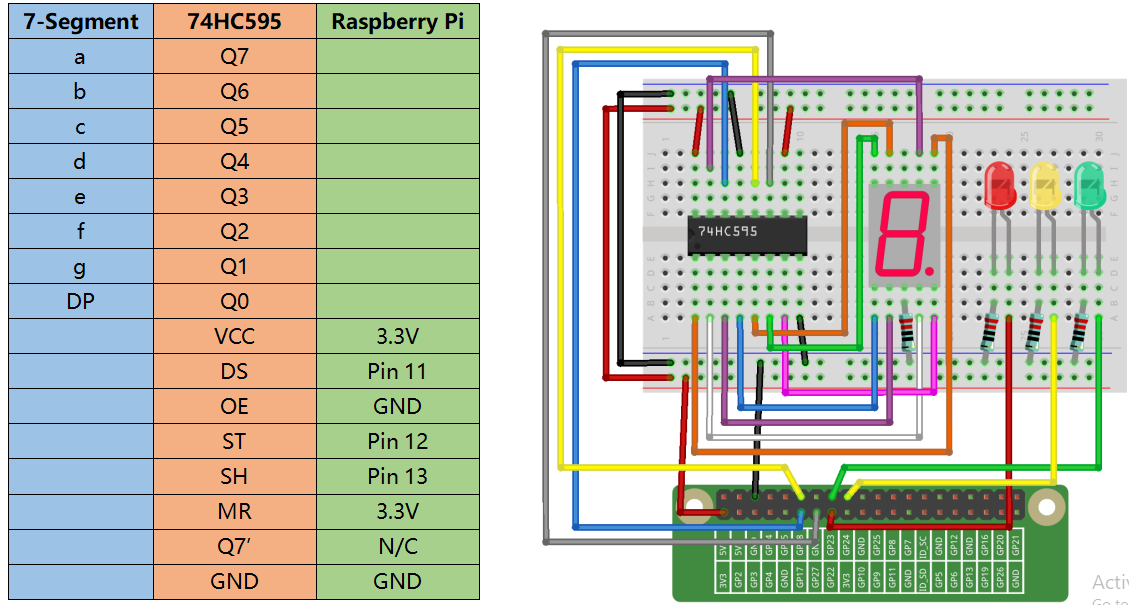## For C Language Users¶

### Command¶

1. Go to the folder of the code.

```cd /home/pi/electronic-kit/for-raspberry-pi/c/Lesson_19_7-segment
```

2. Compile the code.

```gcc 19_7-Segment.c -lwiringPi
```

3. Run the executable file.

```sudo ./a.out
```

You may see 0 to 9 and A to F on the 7-Segment Display.

Note

If it does not work after running, or there is an error prompt: “wiringPi.h: No such file or directory”, please refer to C code is not working?.

### Code¶

```#include <wiringPi.h>
#include <stdio.h>

#define   SDI   0   //serial data input
#define   RCLK  1   //memory clock input(STCP)
#define   SRCLK 2   //shift register clock input(SHCP)

unsigned char SegCode = {0x3f,0x06,0x5b,0x4f,0x66,0x6d,0x7d,0x07,0x7f,0x6f,0x77,0x7c,0x39,0x5e,0x79,0x71,0x80};

void init(void){
pinMode(SDI, OUTPUT);
pinMode(RCLK, OUTPUT);
pinMode(SRCLK, OUTPUT);

digitalWrite(SDI, 0);
digitalWrite(RCLK, 0);
digitalWrite(SRCLK, 0);
}

void hc595_shift(unsigned char dat){
int i;
for(i=0;i<8;i++){
digitalWrite(SDI, 0x80 & (dat << i));
digitalWrite(SRCLK, 1);
delay(1);
digitalWrite(SRCLK, 0);
}

digitalWrite(RCLK, 1);
delay(1);
digitalWrite(RCLK, 0);
}

int main(void){
int i;

if(wiringPiSetup() == -1){ //when initialize wiring failed, print message to screen
printf("setup wiringPi failed !");
return 1;
}

init();

while(1){
for(i=0;i<17;i++){
hc595_shift(SegCode[i]);
delay(500);
}
}
return 0;
}
```

### Code Explanation¶

```10.void init(void){
11.    pinMode(SDI, OUTPUT);
12.    pinMode(RCLK, OUTPUT);
13.    pinMode(SRCLK, OUTPUT);
14.
15.    digitalWrite(SDI, 0);
16.    digitalWrite(RCLK, 0);
17.    digitalWrite(SRCLK, 0);
18.}
```

Initialize pins. Set all control pins of 74HC595 to output mode and initialize them to low level. At the same time, the LEDs are set to output mode, default low level.

```19. void hc595_shift(unsigned char dat)
```

To assign 8 bit value to 74HC595’s shift register.

```22.    for(i=0;i<8;i++){
23.        digitalWrite(SDI, 0x80 & (dat << i));
24.        digitalWrite(SRCLK, 1);
25.        delay(1);
26.        digitalWrite(SRCLK, 0);
27.    }
```

Assign the dat value to SDI(DS) by bits. Then shift them to the shift register by bits. Execute the loop 8 times to shift the 8 bits of dat to the shift register in proper order.

```29.        digitalWrite(RCLK, 1);
30.        delay(1);
31.        digitalWrite(RCLK, 0);
```

Pin RCLK converts from low to high and generates a rising edge, then shifts data from shift register to storage register. Finally the data in the memory register are output to the bus (Q0-Q7).

```45.        for(i=0;i<17;i++){
46.            hc595_shift(SegCode[i]);
47.            delay(500);
48.        }
```

In the for loop, output 16 values from array Segcode[] to 7-Segment Display.

## For Python Language Users¶

### Command¶

1. Go to the folder of the code.

```cd /home/pi/electronic-kit/for-raspberry-pi/python
```

2. Run the code.

```sudo python3 19_7-Segment.py
```

You may see 0 to 9 and A to F on the 7-Segment Display.

### Code¶

Note

You can Modify/Reset/Copy/Run/Stop the code below. But before that, you need to go to source code path like `electronic-kit/for-raspberry-pi/python`. After modifying the code, you can run it directly to see the effect.

```import RPi.GPIO as GPIO
import time

# Set up pins
SDI   = 17
RCLK  = 18
SRCLK = 27

segCode = [0x3f,0x06,0x5b,0x4f,0x66,0x6d,0x7d,0x07,0x7f,0x6f,0x77,0x7c,0x39,0x5e,0x79,0x71]

def setup():
GPIO.setmode(GPIO.BCM)
GPIO.setup(SDI, GPIO.OUT, initial=GPIO.LOW)
GPIO.setup(RCLK, GPIO.OUT, initial=GPIO.LOW)
GPIO.setup(SRCLK, GPIO.OUT, initial=GPIO.LOW)

# Shift the data to 74HC595
def hc595_shift(dat):
for bit in range(0, 8):
GPIO.output(SDI, 0x80 & (dat << bit))
GPIO.output(SRCLK, GPIO.HIGH)
time.sleep(0.001)
GPIO.output(SRCLK, GPIO.LOW)
GPIO.output(RCLK, GPIO.HIGH)
time.sleep(0.001)
GPIO.output(RCLK, GPIO.LOW)

def main():
while True:
# Shift the code one by one from segCode list
for code in segCode:
hc595_shift(code)
time.sleep(0.5)

def destroy():
GPIO.cleanup()

if __name__ == '__main__':
setup()
try:
main()
except KeyboardInterrupt:
destroy()
```

### Code Explanation¶

```12.def setup():
13.    GPIO.setmode(GPIO.BCM)
14.    GPIO.setup(SDI, GPIO.OUT, initial=GPIO.LOW)
15.    GPIO.setup(RCLK, GPIO.OUT, initial=GPIO.LOW)
16.    GPIO.setup(SRCLK, GPIO.OUT, initial=GPIO.LOW)
```

Initialize pins. Set all control pins of 74HC595 to output mode and initialize them to low level.

```19.def hc595_shift(dat):
```

To assign 8 bit value to 74HC595’s shift register.

```20.    for bit in range(0, 8):
21.        GPIO.output(SDI, 0x80 & (dat << bit))
22.        GPIO.output(SRCLK, GPIO.HIGH)
23.        time.sleep(0.001)
24.        GPIO.output(SRCLK, GPIO.LOW)
```

Assign the dat value to SDI(DS) by bits. Then shift them to the shift register by bits. Execute the loop 8 times to shift the 8 bits of dat to the shift register in proper order.

```25.    GPIO.output(RCLK, GPIO.HIGH)
26.    time.sleep(0.001)
27.    GPIO.output(RCLK, GPIO.LOW)
```

Pin RCLK converts from low to high and generates a rising edge, then shifts data from shift register to storage register. Finally the data in the memory register are output to the bus (Q0-Q7).

```32.        for code in segCode:
33.            hc595_shift(code)
34.            time.sleep(0.5)
```

In the for loop, output 16 values from array Segcode [] to 7-Segment Display.

## Phenomenon Picture¶